# Leetcode 84：柱状图中最大的矩形（超详细的解法！！！）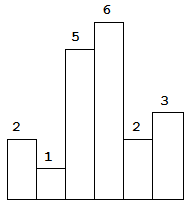输入: [2,1,5,6,2,3]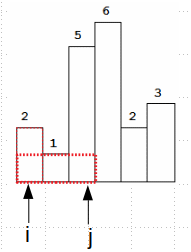class Solution:
def largestRectangleArea(self, heights):
"""
:type heights: List[int]
:rtype: int
"""
if not heights:
return 0
heights_len = len(heights)
res = 0
i, j = 0, 0
while i < heights_len:
min_h = heights[i]
for j in range(i, heights_len):
min_h = min(min_h, heights[j])
res = max(res, min_h*(j - i + 1))
i += 1

return res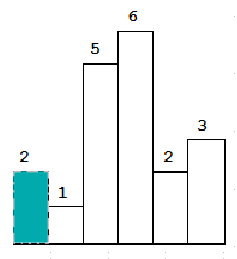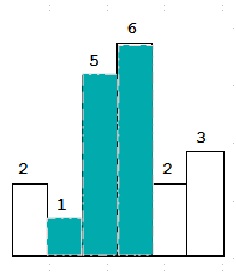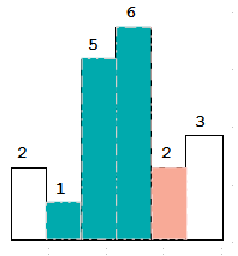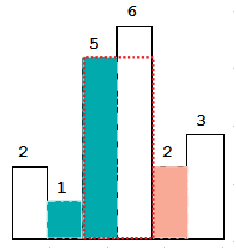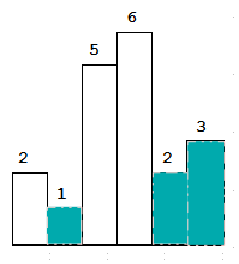class Solution:
def largestRectangleArea(self, heights):
"""
:type heights: List[int]
:rtype: int
"""
stack = list()
res, i = 0, 0
while i < len(heights):
if not stack or (heights[i] >= heights[stack[-1]]):
stack.append(i)
i += 1
else:
k = stack.pop()
res = max(res, heights[k]*((i - stack[-1] - 1) if stack else i))

while stack:
k = stack.pop()
res = max(res, heights[k]*((i - stack[-1] - 1) if stack else i))

return res


class Solution:
def largestRectangleArea(self, heights):
"""
:type heights: List[int]
:rtype: int
"""
stack = [-1]
res = 0
heights.append(-1)

for idx, val in enumerate(heights):
while heights[stack[-1]] > val:
h = heights[stack.pop()]
res = max(res, h*(idx - stack[-1] -1))

stack.append(idx)

return res